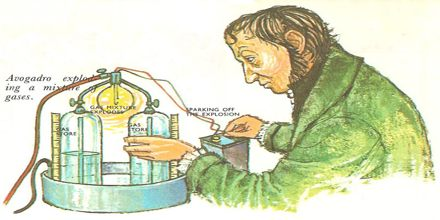Chemistry“Equal volumes of gases under identical temperature and pressure conditions will contain equal numbers of particles (atoms, ion, molecules, electrons, etc.)”

Avogadro’s law is an experimental gas law relating volume of a gas to the amount of substance of gas present. A modern statement of Avogadro’s law is:

Avogadro’s law states that “equal volumes of all gases, at the same temperature and pressure, have the same number of molecules”.

For a given mass of an ideal gas, the volume and amount (moles) of the gas are directly proportional if the temperature and pressure are constant.

which can be written as:

V ∝ n

or, (V/n) = k

where:

V is the volume of the gas
n is the amount of substance of the gas (measured in moles).
k is a constant equal to RT/P, where R is the universal gas constant, T is the Kelvin temperature, and P is the pressure. As temperature and pressure are constant, RT/P is also constant and represented as k. This is derived from the ideal gas law.

Mathematical definition

Avogadro’s law is stated mathematically as:

V/n = k

Where:

V is the volume of the gas(es).
n is the amount of substance of the gas.
k is a proportionality constant.
The most significant consequence of Avogadro’s law is that the ideal gas constant has the same value for all gases.

This means that:

p1 . V1 / T1 . n1 = p2 . V2 / T2 . n2 = constant

Where:

p is the pressure of the gas in the cell
T is the temperature in Kelvin of the gas

One mole of an ideal gas occupies 22.4 liters (dm³) at STP and occupies 24.45 liters at SATP (Standard Ambient Temperature and Pressure = 273K and 1 atm or 101.325 kPa). This volume is often referred to as the molar volume of an ideal gas. Real gases may deviate from this value.